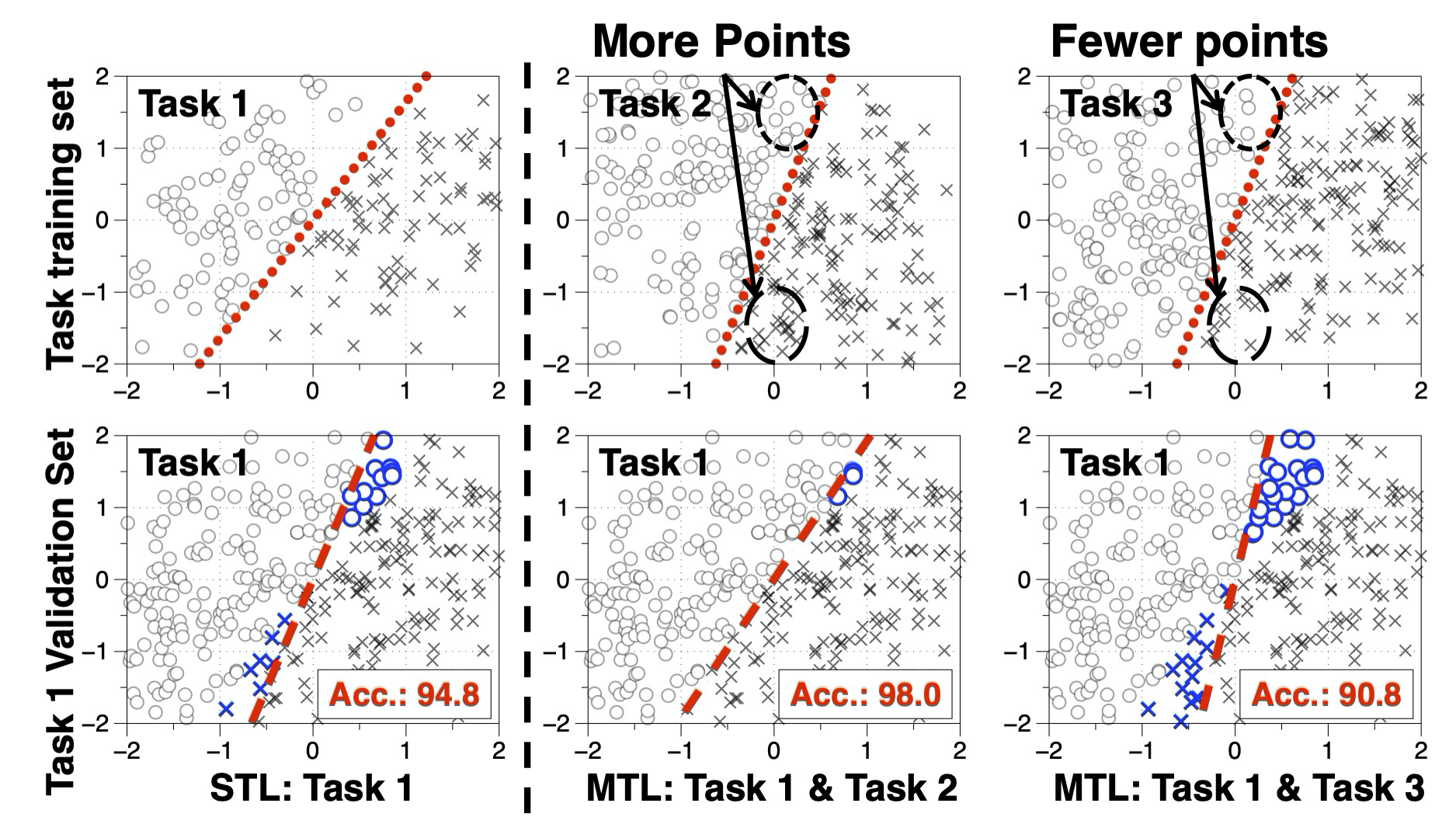# When Multi-Task Learning Works -- And When It Doesn’t

Multi-task learning applied to heterogeneous task data can often result in suboptimal models (or negative transfer in more technical terms). We provide conceptual insights to explain why negative transfer happens. Based on the explanation, we propose methods to improve multi-task training. Based on our work in ICLR’20.

One of the most powerful ideas in deep learning is that sometimes you can take the knowledge learned from one task and apply that knowledge to help another task. For example, maybe a neural network has learned to recognize objects like cats and then help you do a better job finding other objects such as leopards. This is transfer learning, where you learn from task A, and then transfer that to task B. In multi-task learning, you start off simultaneously trying to have one neural network learn several tasks at the same time. And then each of the tasks hopefully helps all the other tasks.

Imagine you are building an autonomous driving vehicle. As a simplified example, the vehicle will need to detect several things, such as traffic lights, road surface marking, other cars on the road and pedestrians. In this example, for each image, your classification task comprises 4 output labels.

As another example, imagine you are building a chatbot for customer service. The model will need to learn to execute a range of different linguistic tasks such as predicting whether the customer’s question reveals a positive or a negative sentiment, how to paraphrase a sentence. To help train a better model, you may also use publicly available data such as Yelp reviews using multi-task learning.

The motivation of our study is that naive multi-task training of neural networks especially on heterogeneous tasks often results in suboptimal models for all the tasks.

To illustrate the phenomenon, we consider three tasks with the same number of data samples where tasks 2 and 3 have the same decision boundary but different data distributions. We observe that training task 1 with task 2 or task 3 can either improve or hurt task 1’s prediction accuracy, depending on the amount of contributing data along the decision boundary! Even though the three tasks have the same linear model, there is no guarantee that multi-task training always helps!Figure 3: Positive vs. Negative transfer is affected by the data, not just the model. See lower right-vs-mid. Task 2 and 3 have the same model (dotted lines) but different data distributions. Notice the difference of data in circled areas.

We have several tasks $X_i,Y_i$ as input for $i=1,...,k$, where $X_i$ corresponds to task features (say a set of images or sentences) and $Y_i$ corresponds to the labels of $X_i$. In the autonomous driving example above, $k=4$, $X_i$ corresponds to the images, and each $Y_i$ corresponds to whether there are stop signs in the image or traffic lights, etc.

One way to solve the problem is by using the so-called hard parameter sharing architecture.

• There is a shared module $B$ that encodes the feature representation for all tasks. For image classification tasks, $B$ can be a CNN such as ResNet. For text classification tasks, $B$ can be pre-trained representations such as BERT.
• Each task has a specific output prediction head $A_i$.
• To train the neural network, you write a loss function that sums over the losses of the predictions for each individual task: $\sum_{i=1}^{k} loss(\hat{Y_i}, Y_i).$

And the loss function is the usual cross-entropy loss for multi-class classification problems. In our study, we ask:

What are the key components to determine whether multi-task learning (MTL) is better than single-task learning (STL)? In response, we identify three components: model capacity, task covariance and optimization scheme.

# Three Factors that Can Result in Negative Transfer

Model capacity. The capacity of the shared module, i.e. its output dimension, plays a fundamental role because, if the shared module is too large, there can be no interference (or transfer of knowledge) between tasks since each of them can be memorized in the shared module which results in zero training loss. If it is too small, there can be destructive interference.

As a rule of thumb, we find that the shared module is best performing when its capacity is smaller than the total capacities of the single-task models. As an example, the right table contains the results for sentiment analysis on six language datasets.

• The best performing MTL model has capacity 100.
• The capacities of the best performing STL models are twice as large on average.

Task covariance. To determine the interference between different tasks, we measure how similar two tasks are by a fine-grained notion called task covariance. As an example, to measure the similarity of two tasks composed of sentences and paragraphs, we encode each sentence into an embedding by replacing each word with a word vector followed by average pooling. By measuring the cosine similarity between two embeddings, we can get information such as the semantic similarities and the use of similar words between the two tasks.

Task covariance measures the alignment of two task input data among their principal directions. Our intuition is that if the principal directions of two task input data are not well-aligned, feeding them into the shared module can cause suboptimal models. To deal with this issue, we propose a covariance alignment algorithm to improve multi-task training by adding an alignment module $R_i$ between task $i$ and the shared module $B$.